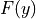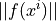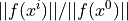# sfepy.solvers.semismooth_newton module¶

class sfepy.solvers.semismooth_newton.SemismoothNewton(conf, **kwargs)[source]

The semi-smooth Newton method.

This method is suitable for solving problems of the following structure:The functionrepresents the smooth part of the problem.

Regular step:Steepest descent step:Although fun_smooth_grad() computes the gradient of the smooth part only, it should return the global matrix, where the non-smooth part is uninitialized, but pre-allocated.

Kind: ‘nls.semismooth_newton’

For common configuration parameters, see Solver.

Specific configuration parameters:

Parameters
semismoothbool (default: True)

If True, use the semi-smooth algorithm. Otherwise a non-smooth equation is assumed (use a brute force).

i_maxint (default: 1)

The maximum number of iterations.

eps_afloat (default: 1e-10)

The absolute tolerance for the residual, i.e..

eps_rfloat (default: 1.0)

The relative tolerance for the residual, i.e..

machepsfloat (default: 2.220446049250313e-16)

The float considered to be machine “zero”.

lin_redfloat (default: 1.0)

The linear system solution error should be smaller than (eps_a * lin_red), otherwise a warning is printed.

ls_onfloat (default: 0.99999)

Start the backtracking line-search by reducing the step, ifis larger than ls_on.

ls_red0.0 < float < 1.0 (default: 0.1)

The step reduction factor in case of correct residual assembling.

ls_red_warp0.0 < float < 1.0 (default: 0.001)

The step reduction factor in case of failed residual assembling (e.g. the “warp violation” error caused by a negative volume element resulting from too large deformations).

ls_min0.0 < float < 1.0 (default: 1e-05)

The minimum step reduction factor.

compute_jacobian(vec_x, fun_smooth_grad, fun_a_grad, fun_b_grad, vec_smooth_r, vec_a_r, vec_b_r)[source]
name = 'nls.semismooth_newton'